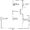# Electronic Circuits January 2008

Note : Answer any FIVE full questions.

1 a. Sketch and explain the circuits of a combination clipper which limit the output between ± 10 V. Assume the diode voltage is 0.7 V.

b.  With neat diagram’and waveforms explain the working of a negative clamper and also write the condition for stiff clamper.

c.  Explain how charge storage is overcome in Schottky diodes.

2 a. Explain small signal operation of amplifiers.

b.  What is the significance of ac emitter resistance in common emitter amplifier?

c.  Calculate the input impedance of the base in Fig. Q 2(c) with p — 150 also draw the acequivalent circuit using 71 model.3 a. With a neat sketch explain the working of a swamped amplifier and derive the expressions for voltage gain and input impedance of the base.

b.  Calculate the output impedance of the amplifier in Fig. Q 3(b).

c.  Write a note on complementary Darlington pair.

4 a. Explain the working of class B push pull emitter follower. Draw its DC and AC load lines.

b. Calculate the efficiency and transistor power dissipation of the class A amplifier shown in Fig. Q 4(b) if the peak to peak output voltage is 18 V and input impedance of the base is 100Q.Fig. Q 4(b)

5 a. Describe the drain curves and Transconductance curve of enhancement mode MOSFET.

c.  With a neat circuit diagram explain CMOS inverter,

6 a. Draw the frequency response of an AC amplifier. Define the terms cut off frequency, mid band gain. Derive the expression for gain in terms of mid band gain and cut off frequencies.

b. OP Amp 74 IC has a mid band gain of 100,000, lower cut off frequency of 10 Hz and roll of rate 20 dB per decade. What is the voltage gain at 10 kHz?

c.  Explain ICVS amplifier.

7 a. Design an OP Amp relaxation oscillator for a frequency of 1 kHz. Also draw the output waveform and waveform across the capacitor.

b.  Write the functional block diagram of IC 555 timer. Explain a stable operation with the circuit diagram. Also draw the output waveform and waveform across the capacitor.

8 a. Define load regulation, line regulation and output resistance for a voltage regulator. For a regulator the measured values are Vnl = 9.91 V, Vfl = 9.81 V Vhl – 9.94 V and Vll — 9.79 V. Calculate the load regulation and line regulation.

b.  What are switching regulators? Explain buck regulator.